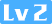【C 陷阱与缺陷】（二）语法陷阱Souleigh ✨935 0 0

### 0. 理解函数声明

``(*(void(*)())0)() ``

``float f, g; ``

``float ((f)); ``

``float ff(); ``

``float *pf; ``

``float *g(), (*h)(); ``

`*g()``(*h)()`是浮点表达式。因为`()`（和`[]`）的优先级高于`*``*g()`也就是`*(g())`：g 是一个函数，该函数返回一个指向浮点数的指针。同理，可以得到 h 是一个函数指针，h 所指向的函数返回值为浮点类型。

``````float (*h)();

(float (*)())p; ``````

``(*fp)(); ``

*fp 就是该指针所指向的函数。ANSI C 标准允许将上式简写为：

``fp(); ``

``void (*fp)(); ``

``(*0)(); ``

``(* (void (*)())0 )(); ``

``````typedef void (*funcptr)();
(*(funcptr)0)(); ``````

### 1. 运算符优先级问题

``````if(FLAG & flags != 0){
...
} ``````

FLAG 是一个已经定义的常量，FLAG 是一个整数，该数的二进制表示中只有某一位是 1，其余的位都为 0 ，也就是 2 的某次幂。为了判断整数 flags 的某一位是否也是 1，并且将结果与 0 作比较，我们写出了上面 if 的判断表达式。

``````if(FLAG & (flags != 0)){
...
} ``````

high 和 low 是两个 0 ~ 15 的数，r 是一个八位整数，且 r 的低 4 位与 low 一致，高 4 位与 high 一致，很自然想到：

``r = high<<4 + low; ``

``r = high<<(4 + low); ``

``r = (high<<4) + low; ``

``r = high<<4 | low; ``

• `a.b.c`的含义是`(a.b).c`而不是`a.(b.c)`

• 函数指针要写成：`(*p)()`，如果写成了`*p()`，编译器会解释为：`*(p())`

• `*p++`会解释为：`*(p++)`而不是`(*p)++`

• 记住两点：

• 任何一个逻辑运算符的优先级低于任何一个关系运算符。
• 移位运算符的优先级比算数运算符要低，但是高于关系运算符。
• 赋值运算符结合方式从右到左，因此：

``a = b = 0; ``

等价于：

``````b = 0;
a = b; ``````
• 关于涉及赋值运算时优先级的混淆：

复制一个文件到另一个文件中：

``````while(c = getc(in) != EOF)
putc(c, out); ``````

但是上式被解释为：

``````while(c = (getc(in) != EOF))
putc(c, out); ``````

关系运算符的结果只有 0 或 1 两种可能。最后得到的文件副本中只包含了一组二进制为 1 的字节流。

### 2. 注意作为语句结束标志的分号

``````if(x[i] > big);
big = x[i]; ``````

``````if(x[i] > big)
big = x[i]; ``````

``````if(x[i] > big) {}
big = x[i]; ``````

``````if( n < 3)
return
logrec.date = x;
logrec.time = x;
logrec.code = x; ``````

``````if( n < 3)
return logrec.date = x;
logrec.time = x;
logrec.code = x; ``````

``````struct logrec{
int date;
int time;
int code;
}
main(){

} ``````

### 3. switch 语句

``````switch(color){
case 1: printf("red");
break;
case 2: printf("blue");
break;
case 3: printf("yellow");
break;
} ``````

``````switch(color){
case 1: printf("red");
case 2: printf("blue");
case 3: printf("yellow");
} ``````

``blueyellow ``

switch 的这种特性，即使它的弱点，也是它的优势所在。

``````case SUBTRACT:
opnd2 = -opnd2;
... ``````

### 4. 函数调用

C 语言要求：在函数调用时，即使函数不带参数，也应该包含参数列表。如果，f 是一个函数：

``f(); ``

``f; ``

### 相关推荐

【C 陷阱与缺陷】（二）语法陷阱
0. 理解函数声明 请思考下面语句的含义： ((void()())0)() 前面我们说过 C 语言的声明包含两个部分：类型和类似表达式的声明符。 最简单的声明符就是单个变量： float f, g; 由于声明符和表达式的相似，我们可以在声明符中任意使用括号： float ((f)); 这个声明的含义是：当对 f 求值时，(
C语言_二进制文件
(https://blog.csdn.net/Ustinian_116/article/details/114783972)二进制文件 其实所有文件最终都是二进制的 想想我们之前printf（）一个整数的时候
C++11新特性学习
1、什么是C+11 ========= C++11标准为C++编程语言的第三个官方标准，正式名叫ISO/IEC 14882:2011 - Information technology -- Programming languages -- C++。在正式标准发布前，原名C++0x。它将取代C++标准第二版ISO/IEC 14882:2003 - Progr
C++创建动态库C#调用（二）
C# TCP与UDP
Http使用端口是80 SMTP使用端口是25 TCP是首选协议，它提供有保证的传输、错误校正和缓冲。 System.Net.Sockets. TcpClient类封装了TCP链接，提供属性字段来控制链接，包括缓冲、缓冲区的大小和超时。 TCP提供很多功能来确保数据的传输，它还提供了错误校正以及当数据丢失或数据包损坏时重新传输的功能。TCP可缓冲传入
C# 生成二维码
1、**添加** **ThoughtWorks.QRCode.dll 引用**  2、代码 using System; using System.Collections.Generic; using System.Linq; using System.Threading.Tasks; using Microsof
C#与.NET
C#从本质上来说，它是一门编程语言。他不是.NET体系的一部分。虽然C#总是用来生成.NET平台的代码。C#语言不能孤立的使用，必须与.NET平台一起使用。这样才能大大提高开发效率。 相对于C++，Java等语言，C#是一种比较新的语言。它是在吸收了C++和Java语言在设计上的优点设计出来的。从一开始C#就被设计为面向对象的开发语言。 .NET Fra
GCC编译 C与C++ C89与C99
1) 最初的 ANSI C 标准 (X3.159-1989) 在 1989 年被批准，并于 1990 年发布。稍后这个标准被接受为 ISO 标准 (ISO/IEC 9899:1990) 。虽然 ISO 标准将 ANSI 标准的某些章节重新编号并变为条款，但是两者实际上并无区别。不论是 ANSI 标准还是 ISO 标准，通常都称之为 C89 ，偶尔也因为发布日
GNU C 与 ANSI C的区别
1.零长度数组 GNU C允许使用零长度数组，定义变长度对象时比较方便 struct var\_data {     int len;     char data\[0\]; }; var\_data的大小仅为一个int型，data是常量地址，data\[index\]是访问其后的内存空间。 struct var\_data \*s = mal
ROS与C++
* 构建工作空间 `**catkin_make**` * 构建Catkin包 `**catkin_create_pkg**` # This is an example, do not try to run this # catkin_create_pkg <package_name> [depend1] [depend2] [dep
ANSI C、ISO C、Standard C联系与区别

Android C、C++与java端3DES互通